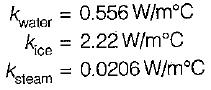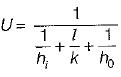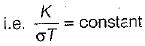Courses

# Test: Basic Concepts of Heat Transfer - 3

## 10 Questions MCQ Test Topicwise Question Bank for Mechanical Engineering | Test: Basic Concepts of Heat Transfer - 3

Description
This mock test of Test: Basic Concepts of Heat Transfer - 3 for Chemical Engineering helps you for every Chemical Engineering entrance exam. This contains 10 Multiple Choice Questions for Chemical Engineering Test: Basic Concepts of Heat Transfer - 3 (mcq) to study with solutions a complete question bank. The solved questions answers in this Test: Basic Concepts of Heat Transfer - 3 quiz give you a good mix of easy questions and tough questions. Chemical Engineering students definitely take this Test: Basic Concepts of Heat Transfer - 3 exercise for a better result in the exam. You can find other Test: Basic Concepts of Heat Transfer - 3 extra questions, long questions & short questions for Chemical Engineering on EduRev as well by searching above.
QUESTION: 1

### Consider the following materials: 1. Carbon 2. Mica 3. Bakelite 4. Fibreglass Q. Which of these materials are good conductors- of heat, but bad conductors of electricity

Solution:

Mica, Bakelite and fibre glass are insulator material and carbon in the form of diamond is the good conductor of heat but bad conductor of electricity

QUESTION: 2

### In which of the following material non-isotropic conductivity is exhibited

Solution:

Wood is considered as orthotropic material since property of grain along the wood is different and across the grain is different.

QUESTION: 3

### Which one of the following have a highest thermal conductivity?

Solution:QUESTION: 4

Thermal diffusivity is

Solution:
QUESTION: 5

Your finger sticks to an ice tray just taken from the refrigerator, Which factor has more effect on this phenomenon?

Solution:

Due to high temperature difference between your finger and the tray, some heat will be transferred to the tray, depending on its capacity for thermal conductivity.
Since the surface of your skin is naturally moisturized (it’s not a function of the air humidity), the loss of heat will cause a thin layer of ice to form on the skin which in turn sticks to the tray. No matter how dry is the air and how cold is the freezer, your finger will not stick to a wooden tray whose thermal conductivity is low. So the most important factor is the thermal conductivity of the tray.

QUESTION: 6

Which one of the following is correct, in context of thermal diffusivity of liquid and gas

Solution:

Since thermal diffusivity, α = k/ρc
(ρC)liquid > (ρC)gas
αgas > αliquid

QUESTION: 7

The unit of thermal diffusivity is

Solution:

Thermal diffusivity, α = K/ρC
Hence its unit = m2/hr or m2/sec
Note that unit of thermal diffusivity and kinematic viscosity is same.

QUESTION: 8

The concept of overall heat transfer is used in the heat transfer in the case of

Solution:

Note that the concept of overall heat transfer is useful in combined mode of heat transfer like conduction and convectionQUESTION: 9

Hot coffee in a cup is allowed to cool, its cooling rate is measured and found to be greater than the value calculated by conduction, convection and radiation measurement. The difference is due to

Solution:
QUESTION: 10

The ratio of thermal conductivity to electrical conductivity is equal to

Solution:

The ratio of thermal conductivity to electrical conductivity is always constant for all the conductors at the given temperature. This is know as Wideman and Frange law.The constant is known as Lorentz number (Lo) whose value is equal to 2.045 x 10-8 W/μK2.